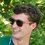# Seemingly simple expected value question

I have no idea how to solve a problem which goes something like this:

"In a neighborhood, 30 people live in 30 different houses. What is the expected number of houses that one must visit in order to find 6 particular people?"

I tried several approaches which gave me strange answers, although I remember one time where I miraculously got something around 23, which seems most reasonable.

Can anyone help me?Note by Finn Hulse
6 years, 2 months ago

This discussion board is a place to discuss our Daily Challenges and the math and science related to those challenges. Explanations are more than just a solution — they should explain the steps and thinking strategies that you used to obtain the solution. Comments should further the discussion of math and science.

When posting on Brilliant:

• Use the emojis to react to an explanation, whether you're congratulating a job well done , or just really confused .
• Ask specific questions about the challenge or the steps in somebody's explanation. Well-posed questions can add a lot to the discussion, but posting "I don't understand!" doesn't help anyone.
• Try to contribute something new to the discussion, whether it is an extension, generalization or other idea related to the challenge.
• Stay on topic — we're all here to learn more about math and science, not to hear about your favorite get-rich-quick scheme or current world events.

MarkdownAppears as
*italics* or _italics_ italics
**bold** or __bold__ bold
- bulleted- list
• bulleted
• list
1. numbered2. list
1. numbered
2. list
Note: you must add a full line of space before and after lists for them to show up correctly
paragraph 1paragraph 2

paragraph 1

paragraph 2

[example link](https://brilliant.org)example link
> This is a quote
This is a quote
    # I indented these lines
# 4 spaces, and now they show
# up as a code block.

print "hello world"
# I indented these lines
# 4 spaces, and now they show
# up as a code block.

print "hello world"
MathAppears as
Remember to wrap math in $$ ... $$ or $ ... $ to ensure proper formatting.
2 \times 3 $2 \times 3$
2^{34} $2^{34}$
a_{i-1} $a_{i-1}$
\frac{2}{3} $\frac{2}{3}$
\sqrt{2} $\sqrt{2}$
\sum_{i=1}^3 $\sum_{i=1}^3$
\sin \theta $\sin \theta$
\boxed{123} $\boxed{123}$

## Comments

Sort by:

Top Newest

There are $\dbinom{30}{6}$ ways in which the $6$ people can be "distributed" amongst the $30$ houses with regards to any sequence we choose to take, each of which is equally likely. Now it's the house that we find the $6$th person in that will determine the tally of houses we've had to check. So say the last person was found in the $24$th house we've checked; there are $\dbinom{23}{5}$ ways we could then have found the other $5$ people before getting to this last house. So the expected value will be

$\dfrac{1}{\dbinom{30}{6}} \displaystyle\sum_{k=5}^{29} (k + 1)\dbinom{k}{5} = \dfrac{186}{7} = 26.57$ to 2 decimal places.

That is, for $5 \le k \le 29,$ if the last person found is in the $(k + 1)$st house, then there were $\dbinom{k}{5}$ ways in which we found the previous five people before getting to this last house, each of which was equally likely. The "weight" of each of these outcomes with respect to the expected value calculation is $(k + 1),$ resulting in the above calculation.

It's interesting to analyze the general discrete function

$E(n,m) = \dfrac{1}{\dbinom{n}{m}} \displaystyle\sum_{k=m-1}^{n-1} (k + 1)\dbinom{k}{m-1}$

representing the expected number of houses that need to be visited to find $m$ people distributed amongst $n$ houses. For $n = 30$ and starting at $m = 1$ we obtain the following results:

$15.5, 20.67, 23.25, 24.8, 25.83, 26.57, 27.13, 27.56, 27.9, 28.18, .....$

To find $15$ people we would expect to have to visit just over $29$ houses.

- 6 years, 2 months ago

Log in to reply

The general form is $\dfrac{m(n+1)}{m+1}$

- 6 years, 2 months ago

Log in to reply

Great! I hadn't seen that formula before. I'll have to see now how to simplify my formula for $E(n,m)$ to obtain yours.

- 6 years, 2 months ago

Log in to reply

Don't ask me why I just saw this now, but...

Your summand is $(k+1)\binom{k}{m-1} = m\binom{k+1}{m},$ and now you can factor out the $m$ to get $E(m,n) = \frac{m}{\binom{n}{m}} \sum_{k=m-1}^{n-1} \binom{k+1}{m}$ and that sum, $\binom{m}{m} + \binom{m+1}{m} + \cdots + \binom{n}{m}$, is well-known to equal $\binom{n+1}{m+1}$.

So $E(m,n) = \frac{m \binom{n+1}{m+1}}{\binom{n}{m}} = \frac{m(n+1)}{m+1}$ as desired.

- 5 years, 4 months ago

Log in to reply

Great! Once you rewrite the summand that way the "hockey-stick" identity does conveniently pop out. Thanks for proving that simplification. :)

- 5 years, 4 months ago

Log in to reply

Thanks man! :D

- 6 years, 2 months ago

Log in to reply

You're welcome. :) The value of $26.57$ was higher than I would have anticipated, but "intuition" is pretty unreliable when it come to probability and expected value questions.

- 6 years, 2 months ago

Log in to reply

A possible (but ultimately wrong) intuition is that "if everyone is equally distributed, then I would expect the 6th person to appear in the last $\frac{1}{6}$ of the houses, so the answer is about 27."

Staff - 6 years, 2 months ago

Log in to reply

Your question is analogous to this one

- 6 years, 2 months ago

Log in to reply

Hmm.

- 6 years, 2 months ago

Log in to reply

×

Problem Loading...

Note Loading...

Set Loading...